# 自然场景文本检测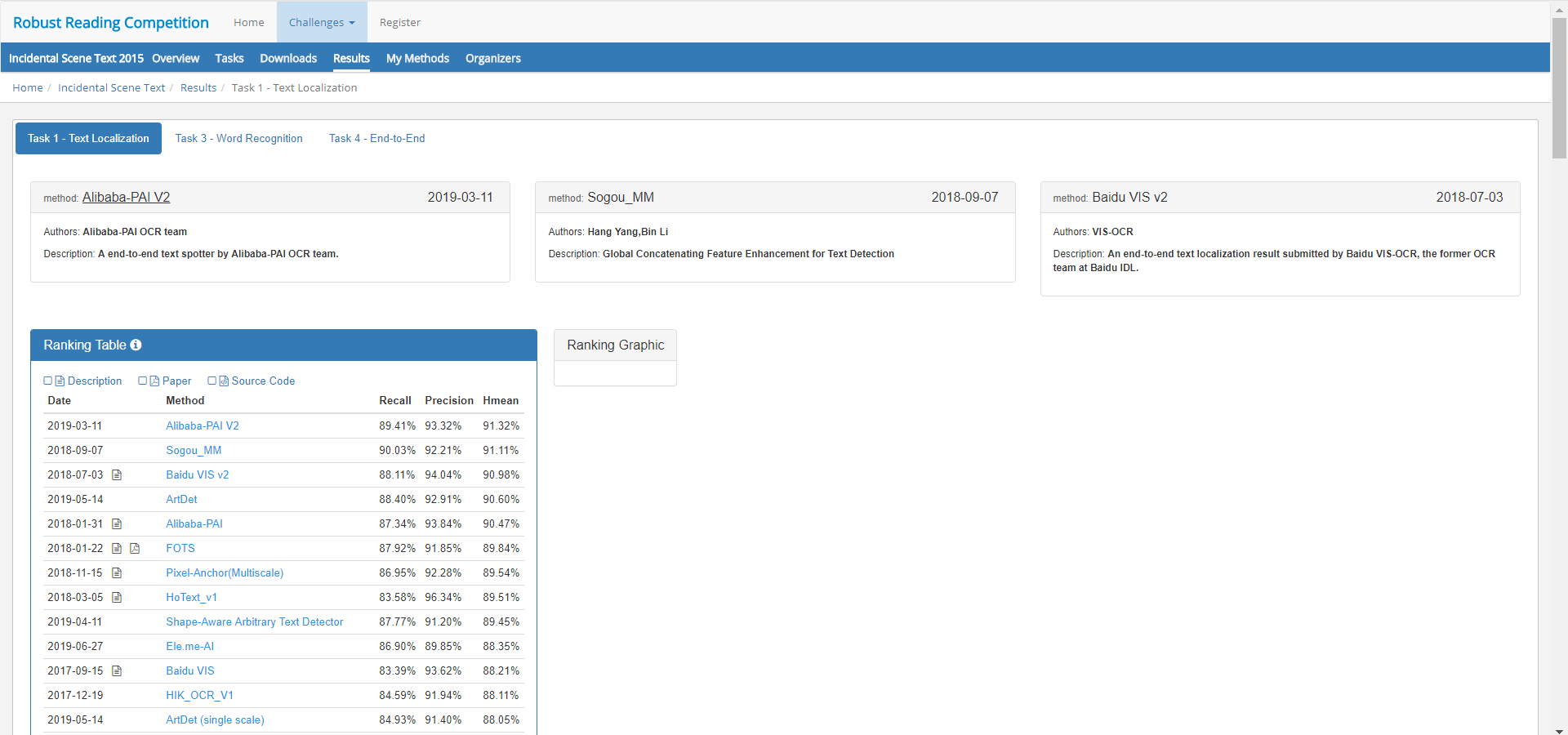# EAST

## 优点

1）步骤简化：传统的文本检测方法和一些基于深度学习的文本检测方法，大多是Multi-stage，在训练时需要对多个Stage调优，这势必会影响最终的模型效果，而且非常耗时。针对上述存在的问题，EAST提出了端到端的文本检测方法，消除中间多个Stage(如候选区域聚合，文本分词，后处理等)，直接预测文本行，其架构就是下图中对应的E部分，跟前面的方法比起来的确少了比较多的过程。（类似于经典的CTPN架构）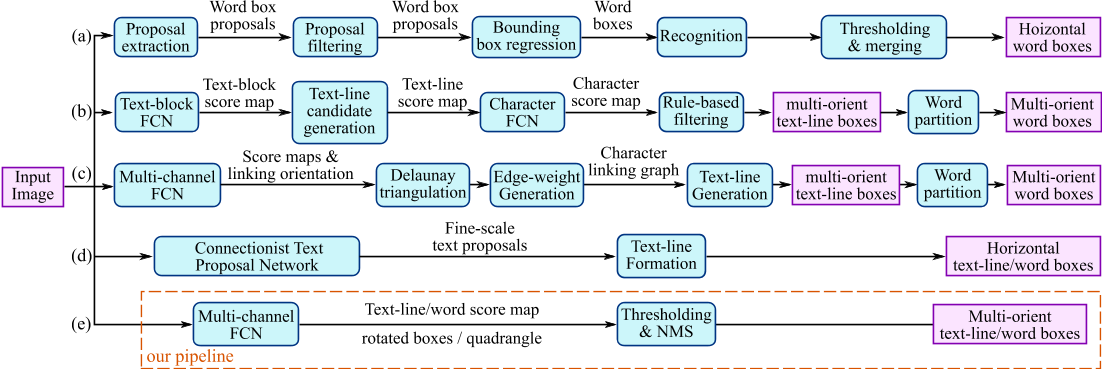2）多方向文本定位：虽然CTPN方法在水平文本的检测方面效果比较好，但是对于竖直文本或者倾斜的文本，该方法的检测就很差，而EAST能支持多方向文本的定位。

## 网络结构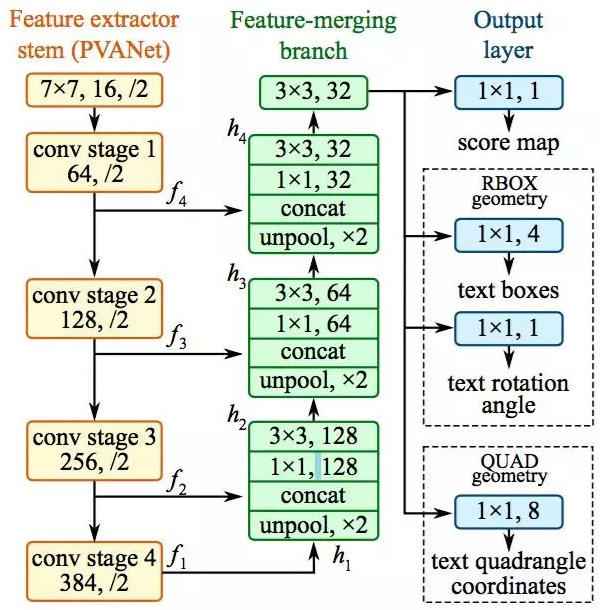## 优点

• 基于Keras，易于阅读和运行
• 基于EAST，一种先进的文本检测算法
• 易于训练模型
• 进行了重大改进，长文本预测更准确

## 网络结构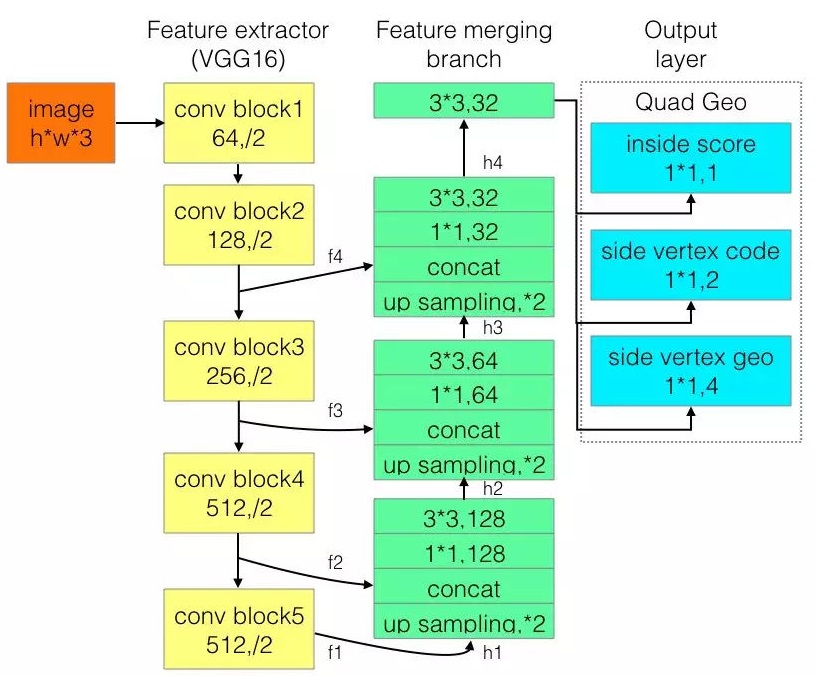`|--data|----test|-------demo 测试样例|-------predict 预测样例|----train|-------ICPR_text_train ICPR MTWI 2018 图文识别挑战赛数据集`
```|--model|----east_model_weights_*.h5 模型权重文件|----vgg16_weights_tf_dim_ordering_tf_kernels_notop.h5 keras下基于tensorflow的VGG16模型notop权重系数h5文件
|--predict 预测|----num.py |----predict.py ```
`|--train|----cfg.py 参数配置|----preprocess.py 数据预处理|----label.py 数据标签生成|----network.py 网络结构定义|----losses.py 损失函数定义|----data_generator.py 训练数据生成|----advanced_east.py 模型训练`

## 数据集

tianchi ICPR dataset - https://pan.baidu.com/s/1NSyc-cHKV3IwDo6qojIrKA（ye9y）- ICPR MTWI 2018挑战赛：网络图像的文本检测

### 标签点

• 按顺序排列四个点，逆时针旋转，且第一个点为左上角点
• 刚开始选择最左边的点，如果最后计算的第二个点的Y比第一个点大，那就让最后一个点做为第一个点，其他点依次右移

• 以最小的X坐标为起点（起名为A）
• 其他三个点和第一个点（A）连线形成夹角，取中间的点为第三个点（起名C）
• 以AC为连线，在AC上方为D，下方为B
• 最后比较AC和BD的斜率，AC>BD，则顺序调整为DABC；AC<BD，则维持ABCD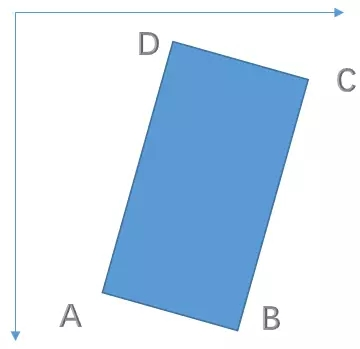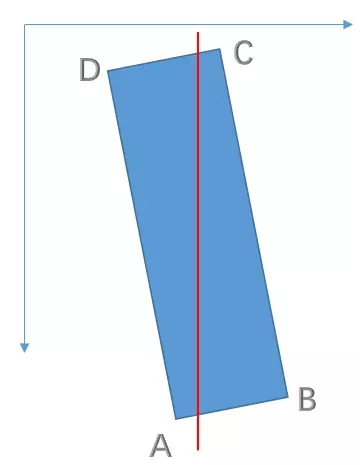• 注意长边的位置：针对上面两幅图，第一张的`long_edge=0,2`，第二张的`long_edge=1,3`

### 标签切边

• 以最短边的0.3进行缩放当做内部点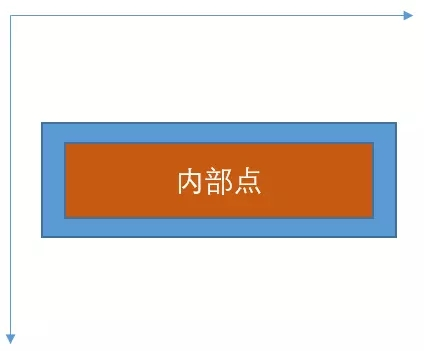• 以最短边的0.6作为头尾点

• 注意：这里头尾都是针对最长边上的操作
• 注意：头和尾是按照标签点的顺序进行的，排在前面为头，排在后面为尾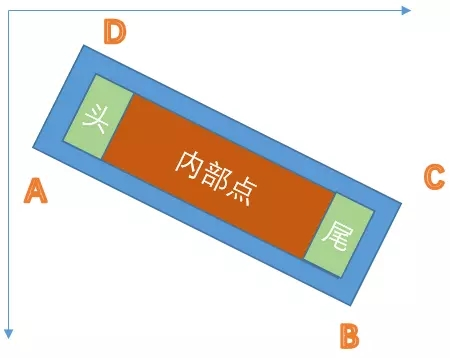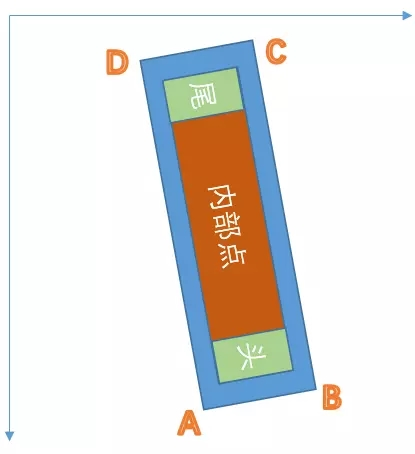## 训练

```1）准备数据集
# 数据集格式 - /ICPR_text_train/train_1000
# cfg.py定义images/labels路径
image_1000/*.jpg
txt_1000/*.txt - X1，Y1，X2，Y2，X3，Y3，X4，Y4，“文本” # X1，Y1，Y2，X2，X3，X4，Y3，Y4 对于文本内容的四边形顶点
）参数配置
cfg.py
）预处理
preprocess.py # 若原始图片过多，可不resize过多图片，[256,736]够了，其在cfg.py更改
）生成标签信息
label.py # 比较耗时
）执行模型训练

### loss计算

```#input : 1*w*h*3
#label : 1*160*160*7(batch,w,h,type)
# loss for inside_score
logits = y_pred[:, :, :, :1]
labels = y_true[:, :, :, :1]
# balance positive and negative samples in an image
beta = 1 - tf.reduce_mean(labels)
# first apply sigmoid activation
predicts = tf.nn.sigmoid(logits)
# log +epsilon for stable cal
inside_score_loss = tf.reduce_mean(
-1 * (beta * labels * tf.log(predicts + cfg.epsilon) +
(1 - beta) * (1 - labels) * tf.log(1 - predicts + cfg.epsilon)))
inside_score_loss *= cfg.lambda_inside_score_loss

# loss for side_vertex_code
vertex_logits = y_pred[:, :, :, 1:3]
vertex_labels = y_true[:, :, :, 1:3]
vertex_beta = 1 - (tf.reduce_mean(y_true[:, :, :, 1:2])
/ (tf.reduce_mean(labels) + cfg.epsilon))
vertex_predicts = tf.nn.sigmoid(vertex_logits)
pos = -1 * vertex_beta * vertex_labels * tf.log(vertex_predicts +
cfg.epsilon)
neg = -1 * (1 - vertex_beta) * (1 - vertex_labels) * tf.log(
1 - vertex_predicts + cfg.epsilon)
positive_weights = tf.cast(tf.equal(y_true[:, :, :, 0], 1), tf.float32)
side_vertex_code_loss = \
tf.reduce_sum(tf.reduce_sum(pos + neg, axis=-1) * positive_weights) / (
tf.reduce_sum(positive_weights) + cfg.epsilon)
side_vertex_code_loss *= cfg.lambda_side_vertex_code_loss

# loss for side_vertex_coord delta
g_hat = y_pred[:, :, :, 3:]
g_true = y_true[:, :, :, 3:]
vertex_weights = tf.cast(tf.equal(y_true[:, :, :, 1], 1), tf.float32)
pixel_wise_smooth_l1norm = smooth_l1_loss(g_hat, g_true, vertex_weights)
side_vertex_coord_loss = tf.reduce_sum(pixel_wise_smooth_l1norm) / (
tf.reduce_sum(vertex_weights) + cfg.epsilon)
side_vertex_coord_loss *= cfg.lambda_side_vertex_coord_loss
return inside_score_loss + side_vertex_code_loss + side_vertex_coord_loss

def smooth_l1_loss(prediction_tensor, target_tensor, weights):
diff = prediction_tensor - target_tensor
abs_diff = tf.abs(diff)
abs_diff_lt_1 = tf.less(abs_diff, 1)
pixel_wise_smooth_l1norm = (tf.reduce_sum(
tf.where(abs_diff_lt_1, 0.5 * tf.square(abs_diff), abs_diff - 0.5),
axis=-1) / n_q) * weights
return pixel_wise_smooth_l1norm

shape = tf.shape(g_true)
delta_xy_matrix = tf.reshape(g_true, [-1, 2, 2])
diff = delta_xy_matrix[:, 0:1, :] - delta_xy_matrix[:, 1:2, :]
square = tf.square(diff)
distance = tf.sqrt(tf.reduce_sum(square, axis=-1))
distance *= 4.0
distance += cfg.epsilon
return tf.reshape(distance, shape[:-1])

if __name__ == '__main__':
x, y = data_generator.gen(1)

### NMS(非极大值抑制)

• 作用：去掉detection任务重复的检测框（不是局部的最大值都去除）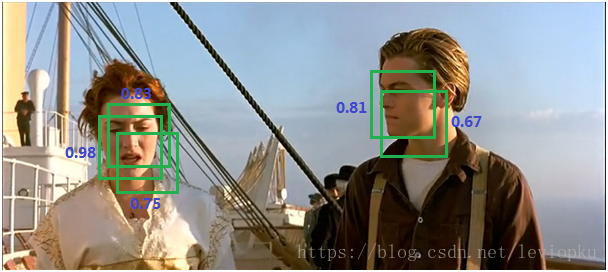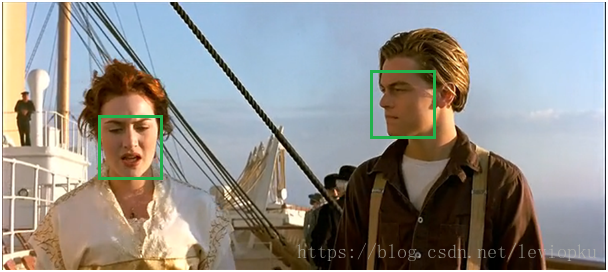```pixel_threshold = 0.9 #内部点阈值(目标点概率)
side_vertex_pixel_threshold = 0.9 #内部头尾点的阈值
trunc_threshold = 0.1 ```

## 预测文字识别分为两个具体步骤:文字的检测和文字的识别,两者缺一不可,尤其是文字检测,是识别的前提条件,若文字都找不到,那何谈文字识别.今天我们首先来谈一下当今流行的文字检测技术有哪些. 文本检测不是一件简 ...

2. 使用Keras基于RCNN类模型的卫星/遥感地图图像语义分割

遥感数据集 1. UC Merced Land-Use Data Set 图像像素大小为256*256,总包含21类场景图像,每一类有100张,共2100张. http://weegee.vision ...

3. OpenCV_contrib里的Text(自然场景图像中的文本检测与识别)

平台:win10 x64 +VS 2015专业版 +opencv-3.x.+CMake 待解决!!!Issue说明:最近做一些字符识别的事情,想试一下opencv_contrib里的Text(自然场景 ...

4. EAST 自然场景文本检测

自然场景文本检测是图像处理的核心模块,也是一直想要接触的一个方面. 刚好看到国内的旷视今年在CVPR2017的一篇文章:EAST: An Efficient and Accurate S ...

5. 基于Text-CNN模型的中文文本分类实战 流川枫 发表于AI星球订阅

Text-CNN 1.文本分类 转眼学生生涯就结束了,在家待就业期间正好有一段空闲期,可以对曾经感兴趣的一些知识点进行总结. 本文介绍NLP中文本分类任务中核心流程进行了系统的介绍,文末给出一个基于T ...

6. 基于Text-CNN模型的中文文本分类实战

Text-CNN 1.文本分类 转眼学生生涯就结束了,在家待就业期间正好有一段空闲期,可以对曾经感兴趣的一些知识点进行总结. 本文介绍NLP中文本分类任务中核心流程进行了系统的介绍,文末给出一个基于T ...

7. 使用Python基于VGG/CTPN/CRNN的自然场景文字方向检测/区域检测/不定长OCR识别

GitHub:https://github.com/pengcao/chinese_ocr https://github.com/xiaofengShi/CHINESE-OCR |-angle 基于V ...

8. 图像文本跨模态细粒度语义对齐-置信度校正机制 AAAI2022

论文介绍:Show Your Faith: Cross-Modal Confidence-Aware Network for Image-Text Matching (跨模态置信度感知的图像文本匹配网 ...

9. 深度学习与计算机视觉(12)_tensorflow实现基于深度学习的图像补全

深度学习与计算机视觉(12)_tensorflow实现基于深度学习的图像补全 原文地址:Image Completion with Deep Learning in TensorFlow by Bra ...

## 随机推荐

1. Python - 工具：将大图切片成小图，将小图组合成大图

训练keras时遇到了一个问题,就是内存不足,将 .fit 改成 .fit_generator以后还是放不下一张图(我的图片是8192×8192的大图==64M).于是解决方法是将大图切成小图,把小图 ...

2. 将本地文件夹同步到github仓库中

参考博客 本地生成rsa密钥 cd ~/.ssh # 查看是否已经生成过密钥 ssh-keygen -t rsa -C "username on github" # -C表示注释 ...

3. svn 同步hook

hook 目录下 cp pre-revprop-change.tmpl pre-revprop-change chmod a+x pre-revprop-change 同步代码初始化 sudo svn ...

4. VMware安装CentOS后无法无法识别网卡的解决方法

请找到安装CentOS虚拟机的安装目录,找到.vmx后缀名的文件,点击鼠标右键选择用记事本打开,在文件的最后一行添加内容:ethernet0.virtualDev = "e1000" ...

5. java 包引入时*会全部加载吗

6. 代码实现程序启动后, 可以从键盘输入接收多个整数, 直到输入quit时结束输入. 把所有输入的整数倒序排列打印

package com.loaderman.test; import java.util.Comparator; import java.util.Scanner; import java.util. ...

7. Python中将（字典，列表等）变量格式化成字符串输出

比如原始的List变量的值是这种: [{"]}] 而想要将其输出为带缩进的,树状的,很漂亮的效果,那么可以通过这样的方法: import json #demoDictList is the ...

8. Session中的方法概述

一.操作实体对象 delete()把持久化或游离转为删除状态 save()把临时状态变为持久化状态(交给Sessioin管理) saveOrUpdate()把临时或游离状态转为持久化状态 update ...

9. "美团"APP学习记录知识点

1.APP进入加载广告视图展示: -(void)initAdvView{ // 判断图片是否已经存在 NSArray *paths = NSSearchPathForDirectoriesInDoma ...

10. C语言基础：递归函数，全局(局)变量

#include <stdio.h>int factorial(int a); int Fibonacci(a);long Hanoi(a); void main(){ } 函数递归调用: ...